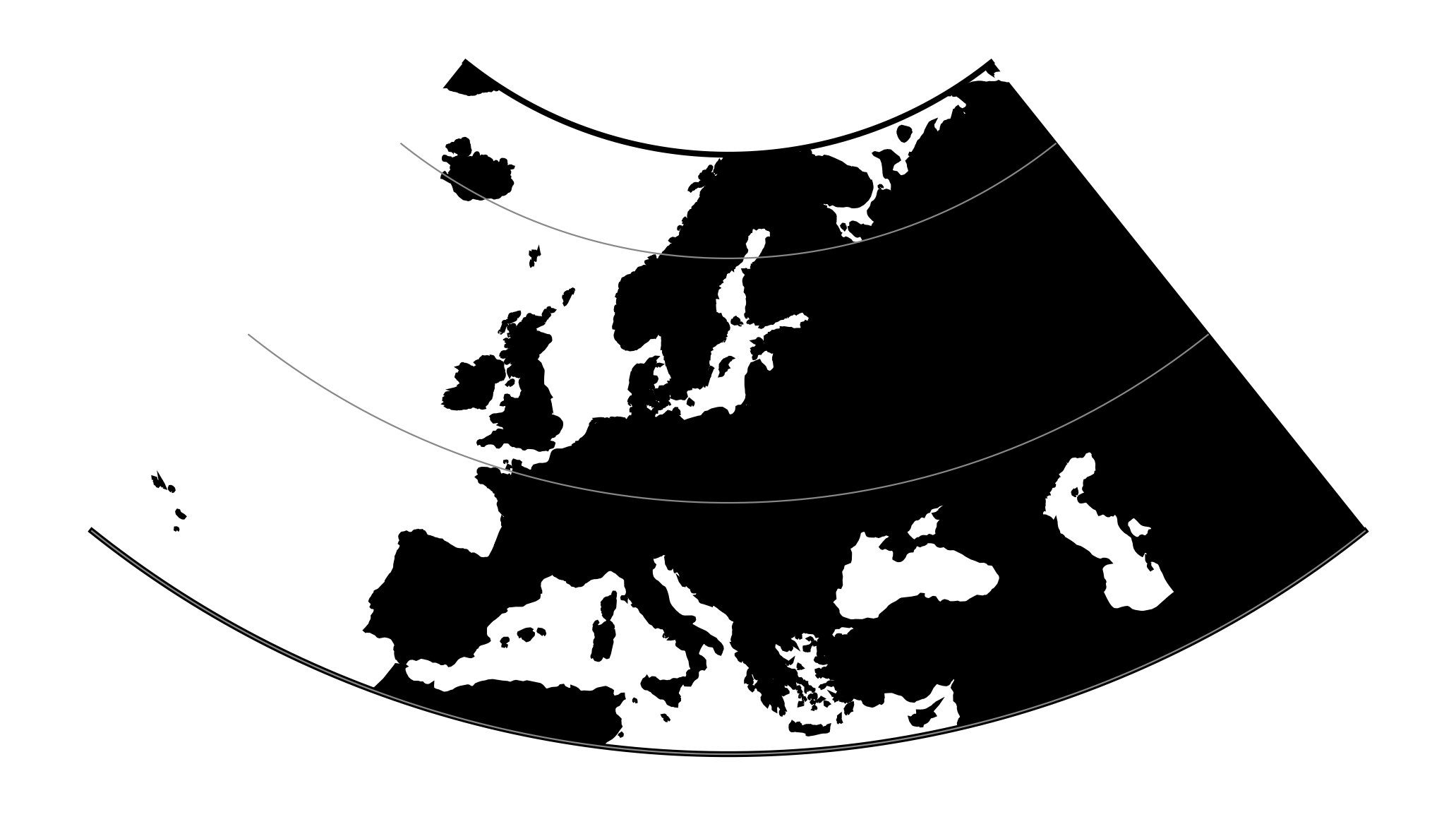# Central Conic¶

New in version 5.0.0.

This is central (centrographic) projection on cone tangent at :option:lat_1 latitude, identical with conic() projection from mapproj R package.

 Classification Conic Available forms Forward and inverse, spherical projection Defined area Global, but best used near the standard parallel Alias ccon Domain 2D Input type Geodetic coordinates Output type Projected coordinatesproj-string: +proj=ccon +lat_1=52 +lon_0=19

## Usage¶

This simple projection is rarely used, as it is not equidistant, equal-area, nor conformal.

An example of usage (and the main reason to implement this projection in proj4) is the ATPOL geobotanical grid of Poland, developed in Institute of Botany, Jagiellonian University, Krakow, Poland in 1970s [Zajac1978]. The grid was originally handwritten on paper maps and further copied by hand. The projection (together with strange Earth radius) was chosen by its creators as the compromise fit to existing maps during first software development in DOS era. Many years later it is still de facto standard grid in Polish geobotanical research.

The ATPOL coordinates can be achieved with with the following parameters:

+proj=ccon +lat_1=52 +lon_0=19 +axis=esu +a=6390000 +x_0=330000 +y_0=-350000


## Parameters¶

### Required¶

+lat_1=<value>

Standard parallel of projection.

### Optional¶

+lon_0=<value>

Longitude of projection center.

Defaults to 0.0.

+R=<value>

Radius of the sphere given in meters. If used in conjunction with +ellps +R takes precedence.

+x_0=<value>

False easting.

Defaults to 0.0.

+y_0=<value>

False northing.

Defaults to 0.0.

## Mathematical definition¶

### Forward projection¶

$r = \cot \phi_0 - \tan (\phi - \phi_0)$
$x = r \sin (\lambda\sin\phi_0)$
$y = \cot \phi_0 - r \cos (\lambda\sin\phi_0)$

### Inverse projection¶

$y = \cot \phi_0 - y$
$\phi = \phi_0 - \tan^{-1} ( \sqrt{x^2+y^2} - \cot \phi_0 )$
$\lambda = \frac{\tan^{-1} \sqrt{x^2+y^2}}{\sin \phi_0}$

## Reference values¶

For ATPOL to WGS84 test, run the following script:

#!/bin/bash
cat << EOF | src/cs2cs -v -f "%E" +proj=ccon +lat_1=52 +lat_0=52 +lon_0=19 +axis=esu +a=6390000 +x_0=330000 +y_0=-350000 +to +proj=longlat
0 0
0 700000
700000 0
700000 700000
330000 350000
EOF


It should result with

1.384023E+01 5.503040E+01 0.000000E+00
1.451445E+01 4.877385E+01 0.000000E+00
2.478271E+01 5.500352E+01 0.000000E+00
2.402761E+01 4.875048E+01 0.000000E+00
1.900000E+01 5.200000E+01 0.000000E+00


Analogous script can be run for reverse test:

cat << EOF  | src/cs2cs -v -f "%E" +proj=longlat +to +proj=ccon +lat_1=52 +lat_0=52 +lon_0=19 +axis=esu +a=6390000 +x_0=330000 +y_0=-350000
24 55
15 49
24 49
19 52
EOF


and it should give the following results:

6.500315E+05 4.106162E+03 0.000000E+00
3.707419E+04 6.768262E+05 0.000000E+00
6.960534E+05 6.722946E+05 0.000000E+00
3.300000E+05 3.500000E+05 0.000000E+00